Name: becca Who is asking: Student Level of the question: Middle Question: can anyone help with how we change to get the same denominator with adding whole number. example:8+7 3/8 6 1/2 + 2 5/6 Hi Becca. The easiest way is to first convert these "mixed fractions" (that's where you have a whole number and a fraction) to "improper fractions" (that's where there is no whole number, but the numerator of the fraction is larger than the denominator). Let me give an example: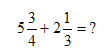Start by converting each mixed fraction to an improper fraction: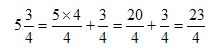and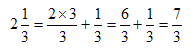So we know now that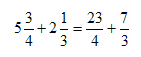The next step is to add these improper fractions. But to do that, we must first find a common denominator. I'll "cross-multiply" (that is, I'll multiply each fraction's top and bottom by the bottom of the other fraction):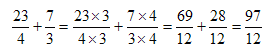The last step is to convert our improper fraction back to a mixed fraction: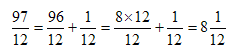I hope this example helps you in your own questions! Stephen La Rocque.